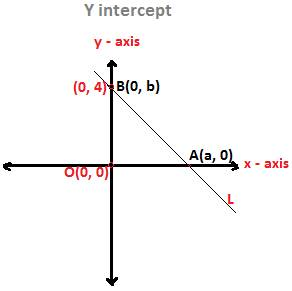## Y-interceptWhat is y intercept?

In this lesson, we will try to understand what is meant by y intercept?

In the above XY Coordinate plane, consider a straight line L.

This line L passes through the point (a, 0) on the x-axis and the point (0, b) on the y-axis.

y intercept definition:

The y coordinate in the point (0, b) on the y-axis through which the line L passes is called the y intercept.

y intercepts exist with respect to a line. They do not exist independently.

i.e. the y coordinate of a point through which no straight line is given to pass does not turn into y intercept, rather it remains just as a y coordinate.

In the above figure, the straight line L passes through the point B (0, b) on the y-axis.

Therefore, the y coordinate b is the y intercept of the line L.

Since the y intercept is basically y coordinate, so it can be both positive and negative.

When the straight line passes through the y-axis from above the origin, then the y intercept is positive, because, y coordinates of points lying on y-axis above the origin are positive.

And again, the y intercept of the line that cuts the y-axis from below the origin is negative, because y coordinates of points lying below the origin are negative.

Thus, y intercepts are both positive and negative.

Now, we will discuss two methods below for finding y intercept of a line L

The following two methods for finding y intercept of a Line L will apply when the slope of the line and a point through which it is passing through are given.

Method-1:

Find the y intercept of a straight line L whose slope is 2 and which passes through the point P (3, 4)

Solution:

Recall from above, what is a y intercept?

It is the y coordinate in the point on the y-axis through which a line L will pass.

So, write the point as B (0, b), in which the y intercept of the Line L is b. Remember, this line L will pass through the point B (0, b).

Now, there are two points B (0, b) and P (3, 4) through which the Line will pass.

Again, slope of Line L is 2, as given.

Applying slope formula on B (0, b) and P (3, 4), we have,

(b – 4)/ (0 – 3) = 2,

b – 4 = -6, b = -6 + 4 = - 2

Method 2:

Alternatively, use equation of a straight line to find y intercept of a line L.

We can use the slope-intercept form of the equation of a straight line L, as since slope and a point through which the line is passing are given.

Now, the equation of a straight line L in slope-intercept form is

y = mx + b,

In this formula, m is slope of the line L and b is the y intercept.

Substitute the coordinates of the point P (3, 4) in the equation of the Line L as it is passing through this point and 2 in slope m.

4 = 2 × 3 + b,

So, b = - 2

X intercept